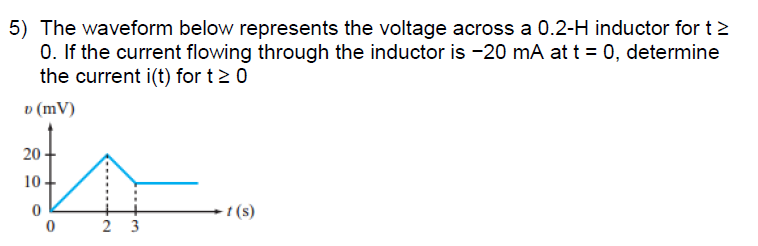Create an Account

Home / Questions / The waveform below represents the voltage across a 02 H inductor for t 0 If the current f...

The waveform below represents the voltage across a 02 H inductor for t 0 If the current flowing through the inductor is

The waveform below represents the voltage across a 0.2-H inductor for t ? 0. If the current flowing through the inductor is ?20 mA at t = 0, determine the current i(t) for t ? 0Jul 27 2020 View more View LessSubscribe To Get Solution